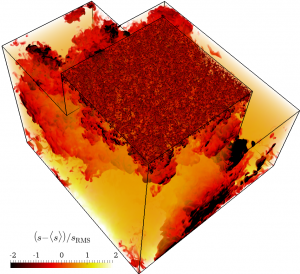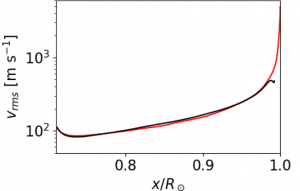# PSTEP Science Nuggets No.19 (20190108)A Comprehensive Numerical simulation from the base of the solar convection zone to the surface.

Hideyuki Hotta (Chiba university)

The outer 30% of the solar interior is called the convection zone and filled with turbulent thermal convection. A prominent feather of the solar convection zone is “strong” stratification. Here “strong” means the density changes orders of magnitude through the convection zone. In the solar case, the density changes by 10^6 and as a result time and spatial scales of the thermal convection changes drastically. At the base of the convection zone, these are one month and 200 Mm, respectively, while these are several minutes and 1 Mm at the surface. Since it is difficult to cover this extreme scale separation in a calculation, the calculation for the solar convection zone was separated to the deep and the shallow parts. On the other hand, recent theories and observations suggest that the thermal convection in the deep calculations overestimate the convection velocity one order of magnitude compared with the real sun. Many studies indicate that the absence of the surface layer which initially drives the thermal convection causes this overestimation.
In this study, we develop schemes of the equation of state and the radiative transfer for treating the physics as precise as possible with maintaining the efficiency in the massive supercomputer. With using the new code R2D2 (Radiation and RSST for Deep Dynamics) with these methods, we for the first time carry out a comprehensive calculation of the whole convection zone from the base to the surface. We also have a calculation without the surface for comparing the structure of the thermal convection between the two cases.
Figure 1 shows the overall structure of the thermal convection with using the entropy perturbation. 1 Mm scale convection at the surface repeatedly merges and constructs the 200 Mm scale convection. Please refer movies in the published paper. Figure 2 compares the convection velocity in the calculations with (red) and without (black) the solar surface. While the calculation with the surface has a larger velocity due to the short timescale radiation cooling, the convection velocities are almost the same between cases in the deep region. In the near surface layer, the mixing occurs efficiently due to the short scale height and the influence disappears in a short distance.
Even when we include the photosphere, the long-standing problem cannot be solved. We need a drastic modification of theory now. We, however, have a scheme for covering the whole convection zone and combinations of the observations and the theories will drastically promote the understanding of the solar convection zone.Figure 1:Snapshot of the entropy perturbation. Horizontal average is subtracted from the entropy value to calculate the perturbation. Then the value is normalized with the horizontal dispersion of the perturbation.Figure 2:The dispersion of the convection velocity against the distance from the solar center. Red and black lines show the result with and without the surface, respectively.

Reference
H. Hotta, H. Iijima, & K. Kusano, “Weak influence of near-surface layer on solar deep convection zone revealed by comprehensive simulation from base to surface”, Science Advances, 5, 1, 2019